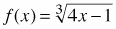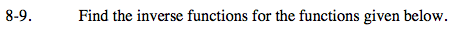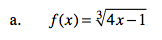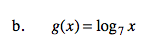Home > A2C > Chapter 8 > Lesson 8.1.1 > Problem8-9

8-9.
1. Find the inverse functions for the functions given below. Homework Help ✎

1.2. g(x) = log7 xSwitch x and f(x) and solve for f(x).

$f^{-1}(x)=\frac{x^{3}+1}{4}$See part (a).